# An Introduction To Angle Sum Property Of A Triangle

In geometry, triangle is one of the basic shapes. Triangle is the smallest polygon. It consists of three edges and three vertices. A triangle with vertices P, Q and R is denoted as ∆PQR. In a triangle, three sides and three angles are referred to as the elements of the triangle. Angle sum property and exterior angle property are the two important attributes of a triangle. What is angle sum property of a triangle? How to prove angle sum property? It is discussed here on.

A triangle consists of interior and exterior angles. Interior angle is defined as the angle formed between two adjacent sides of a triangle. Exterior angle is defined as the angle formed between a side of triangle and an adjacent side extending outward.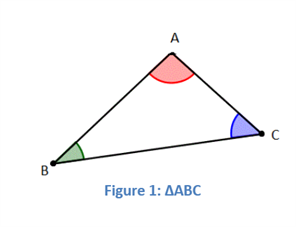For ∆ABC, Angle sum property of triangle declares that

Sum of all the interior angles of the triangle is 180°.

That is,

$$\begin{array}{l}m\angle{A}~+~m\angle{B}~+~m\angle{C}\end{array}$$
=
$$\begin{array}{l}180^{\circ}\end{array}$$

## Proof of Angle Sum Property Theorem

These are the following steps involved to prove angle sum property theorem:

Step 1: Draw a line AB through the vertex P and parallel to the side QR of a triangle PQR.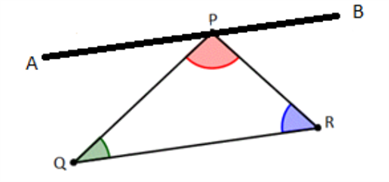Step 2: Now,

$$\begin{array}{l}\angle{APQ}~+~\angle{QPR}~+~\angle{RPB}\end{array}$$
=
$$\begin{array}{l}180^{\circ}\end{array}$$
[Linear Pair Axiom] —— (1)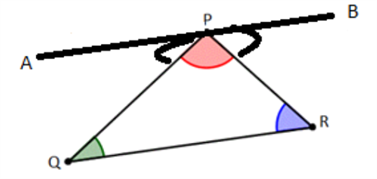Step 3:

$$\begin{array}{l}\angle{APQ}\end{array}$$
=
$$\begin{array}{l}\angle{PQR}\end{array}$$
[Alternate interior angles] —– (2)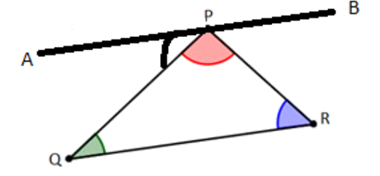Step 4:

$$\begin{array}{l}\angle{RPB}\end{array}$$
=
$$\begin{array}{l}\angle{PRQ}\end{array}$$
[Alternate interior angles] —– (3)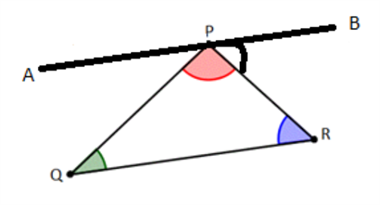Step 5: Substituting

$$\begin{array}{l}∠APQ\end{array}$$
and
$$\begin{array}{l}∠RPB\end{array}$$
in equation 1 by
$$\begin{array}{l}∠PQR\end{array}$$
and
$$\begin{array}{l}∠PRQ\end{array}$$
respectively.

We have thus proved the angle sum property of a triangle.

## Video Lesson on Angle Sum Property of Triangle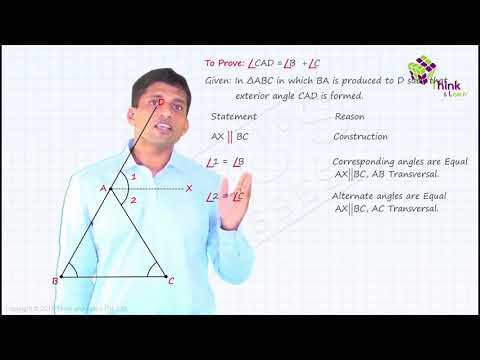For the complete understanding of the topic please visit www.byjus.com or download the BYJU’S– The Learning App.

Test your knowledge on Intro Angle Sum Triangle